# 曲线切线的定义和导数（极限）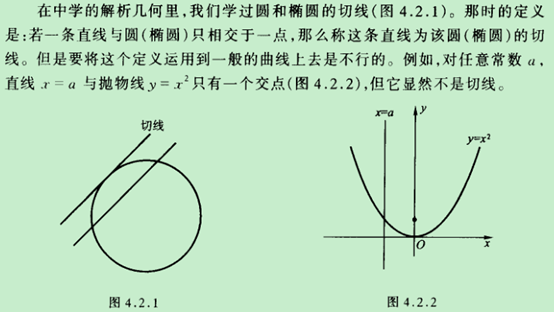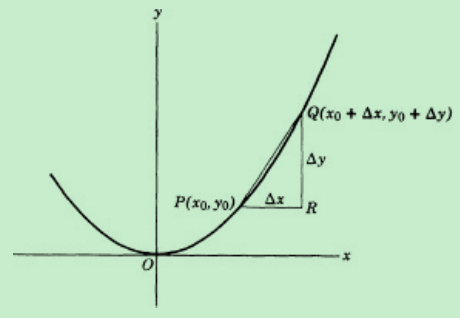$P(x_{0},y_{0})$ $Q(x_{0} + \Delta x,y_{0} + \Delta y)$ 分别是上图曲线上不同的两点（这意味着 $\Delta x \neq 0$ ），Q可以选在P的右边也可以选在左边（这意味着 $\text{Δx}$ 可正可负），称通过PQ的直线为该曲线的一条割线。在 $\text{Δx}$ 不断逼近于0的过程中，点 $Q$ 不断逼近于P，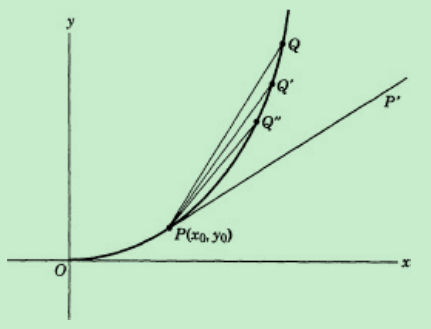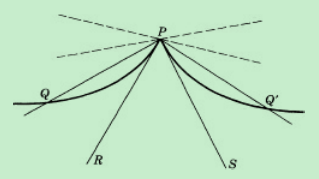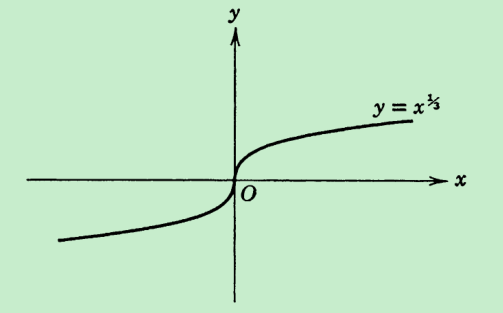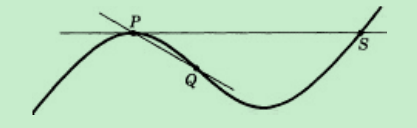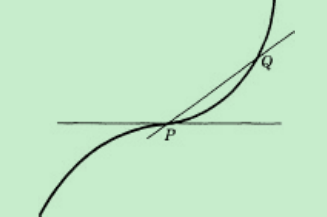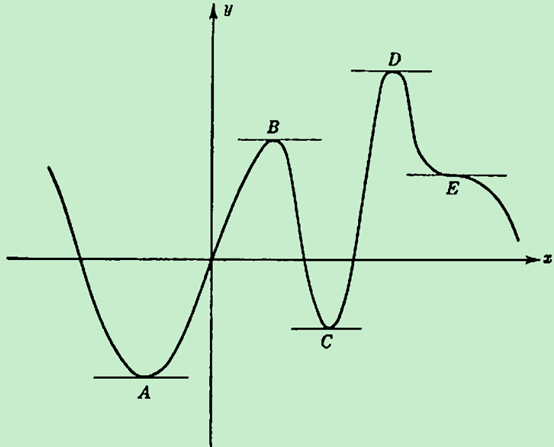1. Morris Kline, Calculus : an intuitive and physical approach, second edition, Chapter 4，Section 1 ↩︎

2. Richard Courant, Fritz John, Introduction to Calculus and Analysis Volume I, Reprint of the 1989 edition, P156 ↩︎

3. Morris Kline, Calculus : an intuitive and physical approach, second edition, Chapter 4，Section 2 ↩︎

4. Richard Courant, Fritz John, Introduction to Calculus and Analysis Volume I, Reprint of the 1989 edition, P156 ↩︎

5. Courant and Robbins, What Is Mathematics? Second Edition, P415 ↩︎

6. Richard Courant, Fritz John, Introduction to Calculus and Analysis Volume I, Reprint of the 1989 edition, Section 3.6, Part b ↩︎

7. Courant and Robbins, What Is Mathematics? Second Edition, P418 ↩︎